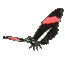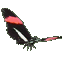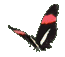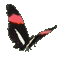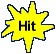Math Help - Multiply One Digit# Times Two Digit# - Game Tips:

- In this game, the first number can be 2 to 7 and the second number can be 10 to 95.

- The question 3 x 47 = ?, can be answered using 3 x (40 + 7) which is 120 + 21 = 141.
- The question 4 x 63 = ?, can be answered using 4 x (60 + 3) which is 240 + 12 = 252.

- Your Game Score is reduced by the number of butterfly hits.

- To slow the game speed repeat tap/click on the word Slider.
- To increase the game speed repeat tap/click on the word Math.
- Speed can also be adjusted with a keyboard's - and + keys.

- Refresh/Reload the web page to restart the game.